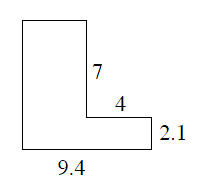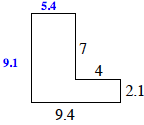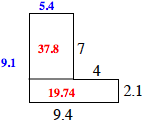### Home > MC1 > Chapter 9 > Lesson 9.2.2 > Problem9-69

9-69.

Find the area and perimeter of the figure at right. All angles are right angles. Show your work.Use the new side labels to help you find both the area and the perimeter of this figure.
Remember that area is the space within a shape and perimeter is the distance around a shape.

To find the area, add the areas of the separate sections, as labeled in red.
$37.8 + 19.74 = 57.54$ square units